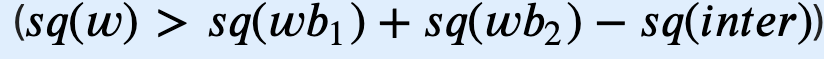# Codeforces Round #587 (Div. 3)（未完太困

A

B

C

CF题解的O(1)办法是求面积，如果下面的不等式成立，那么白纸就有空余。pair<pii, pii> intersect(pii a, pii b, pii c, pii d) {
int lf, rg, up, dn;
lf = max(min(a.x, b.x), min(c.x, d.x));
rg = min(max(a.x, b.x), max(c.x, d.x));
up = min(max(a.y, b.y), max(c.y, d.y));
dn = max(min(a.y, b.y), min(c.y, d.y));

if (rg <= lf || up <= dn) return { {0, 0}, {0, 0} };

return { { lf, dn }, { rg, up } };
}


D

E、F
E询问的范围是1e9，F是1e18。

©️2019 CSDN 皮肤主题: 游动-白 设计师: 上身试试